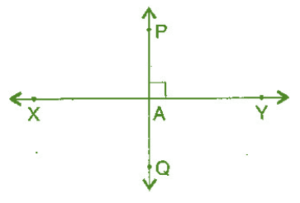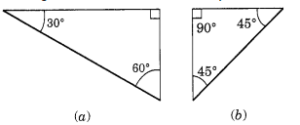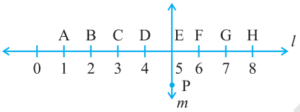# Ncert Class 6 Math Understanding Elementary Shapes Exercise 5.5

Understanding Elementary Shapes Class 6 Ex. 5.5

New Ncert Class 6 Math Free Solution.

Exercise 5.5

Question 1 :- Which of the following are the models for perpendicular lines:
(a) The adjacent edges of a table-top.
(b) The lines of a railway track.
(c) The line segments forming the-letter ‘L’.
(d) The letter V.

Solution 1:-

(a) Perpendicular.
(b) Not perpendicular, railroad tracks have parallel lines running through them. They do not serve as a model for perpendicular lines, therefore.
(c) Perpendicular.
(d) Not perpendicular.

Question 2 :- Let PQ be the perpendicular to the line-segment XY. Let PQ and XY intersect in the point A. What is the measure of ∠PAY?

Solution 2:-
Since PQ ⊥ XY∠PAY = 90°

Question 3 :- There are the two set-squares in your box. What are the measures of the angles that are formed at their corners? Do they have any angle measure that is common?

Solution 3:-
The figures for the two set-squares are shown below:Triangle (a) has measure angles of 30, 60, and 90 degrees.
The measure angles of triangle (b) are 45°, 45° and 90°.
They do have a common angle of 90 degrees.

Question 4 :- Study the diagram. The line l is perpendicular to line m.
(a) Is CE = EG?(b) Does PE bisects CG?
(c) Identify any two line-segments for which PE is the perpendicular bisector.
(d) Are these true?
(i) AC > FG
(ii) CD = GH
(iii) BC < EH

Solution 4:-
(a) Yes,
Since CE and EG both equal two units,
Hence, CE = EG.
(b) Yes, PE bisects CG
(c) Required line segments for which PE is perpendicular bisector are: BH and DF.
(d) (i) True
(ii) True
(iii) True